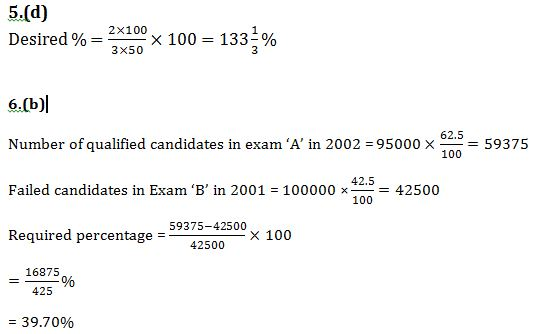# Quant Quiz (Caselet, DI) : 16th October 2018

Quant Quiz to improve your Quantitative Aptitude & Data Interpretation. For upcoming banking exams like SBI PO, SBI Clerk, IBPS PO, IBPS Clerk etc.

Directions (1 to 5): In a college, there are 900 students who are doing PG in any one of the subjects, out of the five different subjects i.e., Zoology, Botany, Mathematics, Physics, and Statistics. The ratio between the boys and the girls among those is 5 : 4 respectively. 20%  of the total girls are doing PG in Zoology and 25% of the total girls are doing PG in Statistics. Total number of studets doing PG in Botany is 220. Total students who are doing PG in Mathematics is 150. Respective ratio between the number of girls and the number of boys doing PG in Statistics is 2 : 3. Twenty percent of the total number of boys are doing PG in Botany. The ratio between the number of girls and boys doing PG in Mathematics is 1 : 2 respectively. There are equal number of boys and girls who are doing PG in Physics. 180 students are doing PG in Zoology.

Q1. What is the total number of students doing PG in Physics and Statistics together?

(a)250

(b)350

(c)300

(d)325

(e)None of these

Q2. What is the respective ratio between two-fifth of  the boys doing PG in Mathematics and one-fourth of  the number of girls doing PG in Botany?

(a)4 : 3

(b)4 : 5

(c)7 : 5

(d)9 : 7

(e)3 : 1

Q3. What is the difference between the boys doing PG in Zoology  and Mathematics and the number of girls doing PG in Mathematics and  Botany?

(a)29

(b)30

(c)32

(d)40

(e)45

Q4. In which PG course  the number of girls is the highest and in which course the number of boys is the lowest (respectively)?

(a) Statistics, Mathematics

(b) Zoology , Botany

(c) Botany, Physics

(d) Mathematics, Zoology

(e) Statistics, Botany

Q5. Twice the Number of girls doing PG in Statistics is what percent of thrice the number of boys doing PG in Physics?Directions (6 to 10): Read both table given below carefully and answer following questions based on these data.

Number of students participated in Exam ‘A’ and Exam ‘B’ from 2000 to 2005.

 Year No. of student (in thousand) A B 2000 85 90 2001 90 100 2002 95 105 2003 110 85 2004 80 85 2005 90 95

Percentage of qualified students in Exam ‘A’ and Exam ‘B’

 Year A (%) B (%) 2000 65 55 2001 60 57.50 2002 62.50 60 2003 67.50 50 2004 55 72.50 2005 57.50 70

Q6. Number of qualified candidates in exam ‘A’ in 2002 is what percent more or less than the failed candidates of Exam ‘B’ in 2001.

(a) 41%

(b) 39.70%

(c) 36.5%

(d) 42.5%

(e) 35.80%

Q7. Maximum number of qualified candidates in exam ‘A’ is recorded in which year ?

(a) 2001

(b) 2002

(c) 2003

(d) 2004

(e) 2005

Q8. Ratio between the total failed student in 2004 in both exam and qualified student of exam ‘A’ in 2000.

(a) 351 : 442

(b) 451 : 342

(c) 442 : 453

(d) 229 : 189

(e) None of these

Q9. What is the average number of students qualified in exam ‘B’ in all year? (Consider nearest integer)

(a) 55938

(b) 54620

(c) 56771

(d) 52940

(e) None of these

Q10. Difference between sum of qualified student of Exam ‘B’ in 2002, 2003, 2004 and sum of qualified students of exam ‘A’ in 2001, 2003, 2005.

(a) 11350

(b) 12455

(c) 13775

(d) 12875

(e) 14780